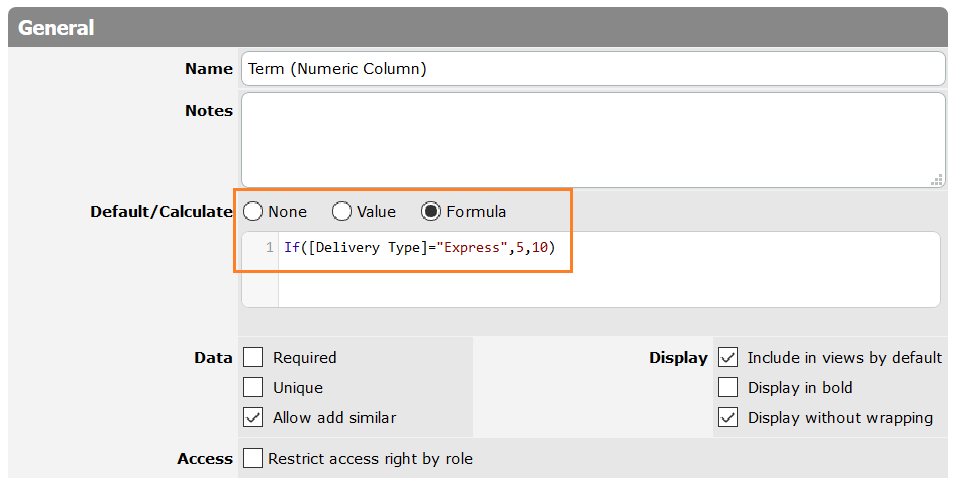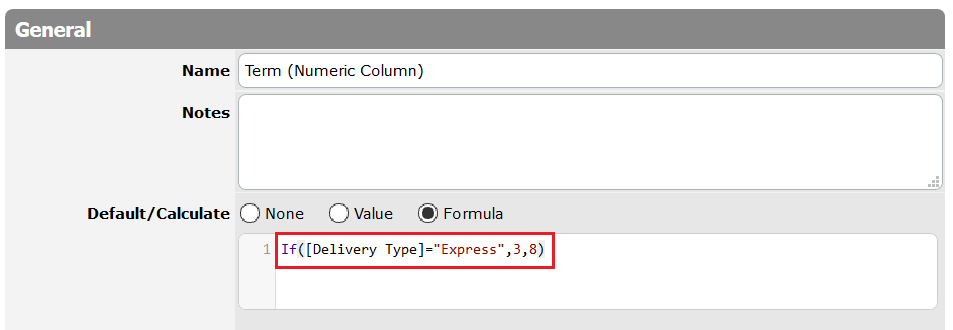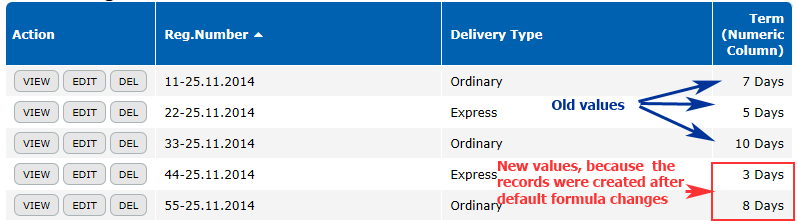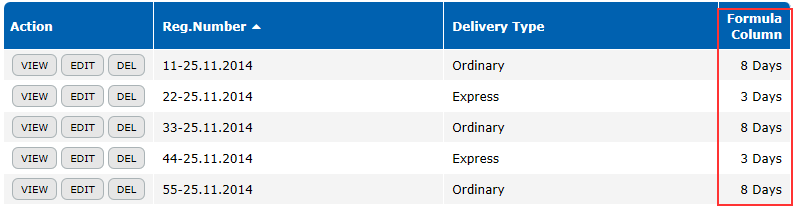# Default/Calculate vs Formula-Column

TeamDesk allows users to create Formula-Columns with formula values. On the other hand, you can use formulas for Default value calculation in columns with simple values (for example the numeric or text type columns).

Let’s consider the difference and define what variant meets your needs better.

## Default/Calculate Formula option

This option is available in the columns containing simple values (for example, the numeric or text type). There is the `Default/Calculate` field with the `Formula` option, where a formula can be entered. According to this formula the column default value is calculated, when a new record is created or when you edit values of columns used in this formula.

Imagine that you’ve created the "Deliveries" table, there are three columns:

"Reg.Number" storing the number of the delivery

"Delivery Type" comprising two choices: Express and Ordinary

"Term (Numeric column)" - where the term of delivery are stored. Each term depends on the Delivery Type value.

The Express delivery term is 5 days, the Ordinary - 10 days.

You can specify the formula in the default/calculate field of the "Term (Numeric column)".

`If ([Delivery Type] = "Express", 5, 10)`

It means if the "Delivery Type" value is "Express", the Term is 5 days, otherwise 10 days.As a result, when record is created, the default value is assigned to the "Term (Numeric column)".If you agreed with a client the other term, you can edit the "Terms (Numeric Column)" and specify any other value.Also if you change your company standards and now the Express delivery is 3 days and the Ordinary is 8 days, you can change the default/calculate formula accordingly:

`If ([Delivery Type] = "Express", 3, 8)`Please note, if some records had been created before the formula was changed, the Term values of such "old" records are not recalculated.## Formula-Column

If you create the column of the Formula type, for example Formula-Numeric type. The column values can’t be changed/edited by a user in the edit mode, only if the values of the columns used as formula variables are changed.

Let’s create the Formula numeric column comprising the formula:

`If ([Delivery Type] = "Express", 5, 10)`Also, if you change the formula, all values will be changed according to the new formula. For example, you changed the formula to the following:

`If ([Delivery Type] = "Express", 3, 8)`